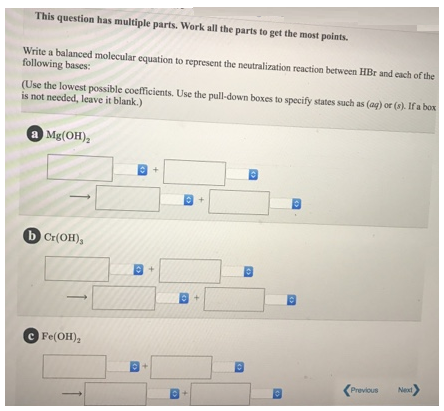# Problem: This question has multiple parts. Work on all the aprts to get the most points.Write a balanced molecular equation to represent the neutralization reaction between HBr and each of the following bases: (Use the lowest possible coefficients. Use the pull-down boxes to specify states such as (aq) or (s). If a box is not needed, leave it blank.) a. Mg(OH)2 b. Cr(OH)3 c. Fe(OH)2

###### FREE Expert Solution
94% (200 ratings)###### Problem Details

This question has multiple parts. Work on all the aprts to get the most points.

Write a balanced molecular equation to represent the neutralization reaction between HBr and each of the following bases: (Use the lowest possible coefficients. Use the pull-down boxes to specify states such as (aq) or (s). If a box is not needed, leave it blank.)

a. Mg(OH)2

b. Cr(OH)3

c. Fe(OH)2